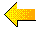Index to site

Stat Labs: Mathematical Statistics through Applications uses a model we have developed for teaching mathematical statistics through in-depth case studies.

Traditional statistics texts have many small numerical examples in each chapter to illustrate a topic in mathematical statistics. Here, we instead make a case study the centerpiece of each chapter. The case studies, which we call labs, raise interesting scientific questions, and by figuring out how to answer these questions we develop statistical theory.

The labs are substantial exercises. Data are provided to address the questions; the solutions are nontrivial and leave room for different analyses. They provide the framework and motivation for the topics in mathematical statistics and they help students develop statistical thinking.

This approach integrates mathematical statistics and applied statistics in a way not commonly encountered in an undergraduate text.

The Student
The book is intended for a course in mathematical statistics for juniors and seniors. We assume that students have had one year of calculus, including Taylor series, and a course in probability. We do not assume students have experience with statistical software so we incorporate lessons on how to use the software into our course.

Statistical Topics
This book covers the standard topics found in most mathematical statistics texts.

 Chapter Main Topic Additional topics 1 - Infant health descriptive statistics quantile plots normal approximation 2 - Video games simple random sampling confidence intervals bootstrap 3 - Radon in households stratified sampling optimal allocation parametric bootstrap 4 - Patterns in DNA estimation and testing maximum likelihood goodness-of-fit tests 5 - Taste testing experiment design of experiments contingency tables chi-square tests of homogeneity & independence 6 - HIV in hemophiliacs Poisson counts and rates confidence intervals for rates Mantel-Haenszel test 7 - Crab growth patterns regression correlation prediction residuals 8 - Instrument calibration Simple linear models replicate measurements transformations confidence bands 9 - Voting behavior ecological regression weighted regression 10 - Infant health multiple regression model checking geometry of least squares indicator variables 11 - Genetically altered mice analysis of variance quantile plots ANOVA table balanced and unbalanced designs 12 - Helicopter design response surface analysis factorial design interactionsAbout Stat Labs How to Use Stat Labs Stat Labs Data Software To Order